## General Question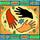# Algebra question - not homework!

Asked by bewailknot (2899) May 7th, 2012

The problem has always bothered me (I took this class 24 years ago and still remember it). I was able to answer it correctly using my head, but I couldn’t “show” my work, so only got partial points.

“The sum of the squares of the first and third of three consecutive odd integers is 106. Find the integers.” (I know they are 5 and 9)

Since the problem started with “sum of squares” that was the formula I tried to use, but I couldn’t make it work out. Can you help me put this to rest?

Observing members: 0Composing members: 0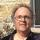Couldn’t you imagine you are dealing with a triangle with a hypotenuse of 106. Make a small table of all integers and their squares and test that each pair of consecutive to see which pair satisfies the Pythagorean theorem. Does that help?

Dr_Lawrence (19747)“Great Answer” (1) Flag as…Wouldn’t that be geometry?

What I did to solve it was make a short list of odd numbers (1, 3, 5, 7, 9). From that point it was easy to see that:
1 and 5 couldn’t work – 1 times 1 is 1, 5 times 5 is 25, 1 plus 25 is 26.
3 and 7 couldn’t work – 3 times 3 is 9, 7 times 7 is 49, 9 plus 49 is 58.
Then 5 and 9, and they did work – 5 times 5 is 25, 9 times 9 is 81, 25 plus 81 is 106.

bewailknot (2899)“Great Answer” (1) Flag as…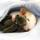An odd integer is of the form 2n+1 for some integer n. Three consecutive odd integers are then 2n+1, 2n+3, and 2n+5. The sum of the squares of the first and third is (2n+1)^2+(2n+5)^2, so the equation is (2n+1)^2+(2n+5)^2 = 106. Simplified, this is n^2+3n-10 = 0 = (n+5)(n-2). The answers for n are -5 and 2, and the odd integers are (-9, -7 -5) or (5, 7, 9). (2*(-5) +1)^2 + (2*(-5)+5)^2 = (-9)^2+(-5)^2 = 81+25 = 106 and (2*2+1)^2+(2*2+5)^2 = 5^2+9^2 = 25+81 = 106.

ratboy (15167)“Great Answer” (5) Flag as…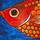Or…....
If x is one of the digits then the other is (x+4)
So the algebraic equation is x^2 + (x+4)^2 = 106
Therefore x is 5 ( or -9 if we include negative solutions)

flutherother (32689)“Great Answer” (5) Flag as…@flutherother, thanks for making me look like an idiot—I didn’t see that the oddness was a red herring.

ratboy (15167)“Great Answer” (1) Flag as…@ratboy No offence meant. There is more than one way to skin a cat.

flutherother (32689)“Great Answer” (0) Flag as…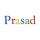Another way, solve using (x-2), x, (x+2) as three numbers. Then, x = +/- 7. Sum of 1st and 3rd terms = 106 for both positive and negative valus. So, three numbers can be either 5,7,9 or -5,-7,-9.

prasad (3859)“Great Answer” (0) Flag as…@flutherother, my remark was a compliment.

ratboy (15167)“Great Answer” (0) Flag as…Thanks all for giving me some insight.

bewailknot (2899)“Great Answer” (0) Flag as…or